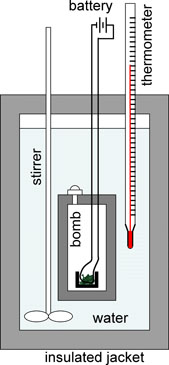## Constant-Volume Calorimetry

#### Learning Objective

• Describe how a bomb calorimeter works

#### Key Points

• A bomb calorimeter is used to measure the change in internal energy, $\Delta U$ , of a reaction. At constant volume, this is equal to qV, the heat of reaction.
• The calorimeter has its own heat capacity, which must be accounted for when doing calculations.

#### Terms

• bomb calorimeterA bomb calorimeter is a type of constant-volume calorimeter used in measuring the heat of combustion of a particular reaction.
• calorieThe amount of energy needed to raise the temperature of 1 gram of water by 1 °C. It is a non-SI unit of energy equivalent to approximately 4.18 Joules. A Calorie (with a capital C) = 1000 calories.

## The Bomb Calorimeter

Bomb calorimetry is used to measure the heat that a reaction absorbs or releases, and is practically used to measure the calorie content of food. A bomb calorimeter is a type of constant-volume calorimeter used to measure a particular reaction’s heat of combustion. For instance, if we were interested in determining the heat content of a sushi roll, for example, we would be looking to find out the number of calories it contains. In order to do this, we would place the sushi roll in a container referred to as the “bomb”, seal it, and then immerse it in the water inside the calorimeter. Then, we would evacuate all the air out of the bomb before pumping in pure oxygen gas (O2). After the oxygen is added, a fuse would ignite the sample causing it to combust, thereby yielding carbon dioxide, gaseous water, and heat. As such, bomb calorimeters are built to withstand the large pressures produced from the gaseous products in these combustion reactions.Bomb calorimeterA schematic representation of a bomb calorimeter used for the measurement of heats of combustion. The weighed sample is placed in a crucible, which in turn is placed in the bomb. The sample is burned completely in oxygen under pressure. The sample is ignited by an iron wire ignition coil that glows when heated. The calorimeter is filled with fluid, usually water, and insulated by means of a jacket. The temperature of the water is measured with the thermometer. From the change in temperature, the heat of reaction can be calculated.

Once the sample is completely combusted, the heat released in the reaction transfers to the water and the calorimeter. The temperature change of the water is measured with a thermometer. The total heat given off in the reaction will be equal to the heat gained by the water and the calorimeter:

$q_{rxn}=-q_{cal}$

Keep in mind that the heat gained by the calorimeter is the sum of the heat gained by the water, as well as the calorimeter itself. This can be expressed as follows:

$q_{cal}=m_{water}C_{water}\Delta T+C_{cal}\Delta T$

where Cwater denotes the specific heat capacity of the water ($1 \frac{cal}{g ^{\circ}C}$), and Ccal is the heat capacity of the calorimeter (typically in $\frac{cal}{^{\circ}C}$). Therefore, when running bomb calorimetry experiments, it is necessary to calibrate the calorimeter in order to determine Ccal.

Since the volume is constant for a bomb calorimeter, there is no pressure-volume work. As a result:

ΔU=qV

where ΔU is the change in internal energy, and qV denotes the heat absorbed or released by the reaction measured under conditions of constant volume. (This expression was previously derived in the “Internal Energy and Enthalpy” section.) Thus, the total heat given off by the reaction is related to the change in internal energy (ΔU), not the change in enthalpy (ΔH) which is measured under conditions of constant pressure.

The value produced by such experiments does not completely reflect how our body burns food. For example, we cannot digest fiber, so obtained values have to be corrected to account for such differences between experimental (total) and actual (what the human body can absorb) values.